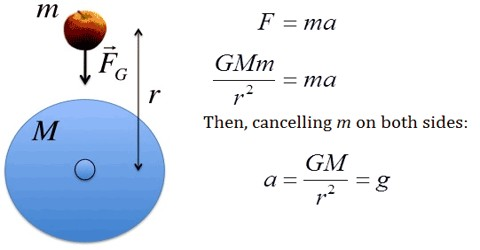# Variation of Acceleration due to Gravity

Variation of Acceleration due to Gravity

We have learnt from the law of gravitation that the value of acceleration due to gravity (g) does not depend on the mass (m) of the body. Value of g depends on the distance of the point from the centre of the earth. From this we can conclude that at a particular position of the earth’s surface value g remains constant, but varies with different locations. Consider the deviation of g when a body moves distance upward or downward from the surface of earth. Let g be the value of acceleration due to gravity at the surface of earth and g’ at a height h above the surface of earth.

Taking mass of the Earth, M = 5.983 x 1024 and Radius, R = 6.36 x 106 m, value of g on Earth’s surface,

g = [(6.657 x 10-11 Nm2kg-2) x (5.983 x 1024 kg) / (6.36 x 106 m)]

= 9.8465 ms-2

On the surface of the earth value of g is more. Again at the equator its value is more than that at the poles. It is zero at the centre of the earth.